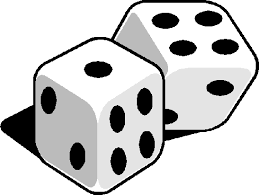# DIE AND COINS

Probability Level 4Alice throws a die. If she gets a 5 or a 6, she tosses three coins and notes the number of heads. Out of these three coins, one is a two-headed-coin and the rest of the two are biased coins each showing heads 75% of the time.

If she gets 1,2,3, or 4, she tosses two coins, one biased (showing heads 75% of the time) and one fair coin, noting whether a head or a tail is obtained.

After Alice throws the die once, she obtains exactly one head. Then if the probability that she threw 1,2,3 or 4 with the die is $\frac{m}{n}$ where $m$ and $n$ are coprime positive integers, what is the value of $m+n?$

×